How the kinetic molecular model of matter helps differentiate various states of matter?

Difficulty: Hard

Kinetic Molecular Model of Matter:

1. The matter is made up of particles called molecules.
2. The molecules remain in continuous motion.
3. Molecules attract each other.

Characteristics of Molecular Model of Matter:

The kinetic molecular model is used to explain the three states of matter - solid, liquid, and gas.

a. Solids:

1. Solids such as stone, metal spoons, pencils, etc. have fixed shapes and volumes.
2. Their molecules are held close together by strong forces of attraction.
3. Molecules of solids vibrate about their mean positions but do not move from place to place.

b Liquids:

1. The distances between the molecules of a liquid are more than in solids. Thus, attractive forces between them are weaker.
2. Like solids, molecules of a liquid also vibrate about their mean position but are not rigidly held with each other.
3. Due to the weaker attractive forces, they can slide over one another. Thus, liquids can flow.
4. The volume of a certain amount of liquid remains the same but because it can flow hence, it attains the shape of a container in which it is put.

c. Gases:

1. Gases such as air have no fixed shape or volume. They can be filled in any container of any shape.
2. Their molecules have random motion and move with very high velocities. In gases, molecules are much farther apart than solids or liquids. Thus, gases are much lighter than solids and liquids.
3. They can be squeezed into smaller volumes.
4. The molecules of a gas are constantly striking the walls of a container. Thus, a gas exerts pressure on the walls of the container. (P ∝E)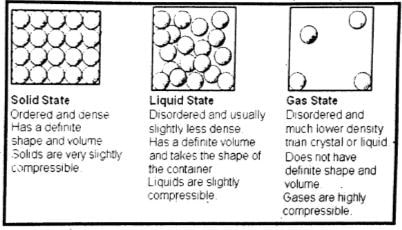d. Plasma – The fourth state of matter:

1. Production/Formation of Plasma:

The kinetic energy of gas molecules goes on increasing if a gas is heated continuously. This causes the gas molecules to move faster and faster. The collisions between atoms and molecules of the gas become so strong that they tear off the atoms. Atoms lose their electrons and become positive ions. This ionic state of matter is called plasma. Plasma is also formed in gas discharge tubes when an electric current passes through these tubes.

2. Plasma is called the fourth state of matter in which a gas occurs in its ionic state. Positive ions and electrons get separated in the presence of electric or magnetic fields.

3. Plasma also exists in neon and fluorescent tubes when they glow.

4. Most of the matter that fills the universe is in the plasma state.

5. In stars such as our Sun, gases exist in their ionic state.

6. Plasma is a highly conducting state of matter. It allows electric current to pass through it.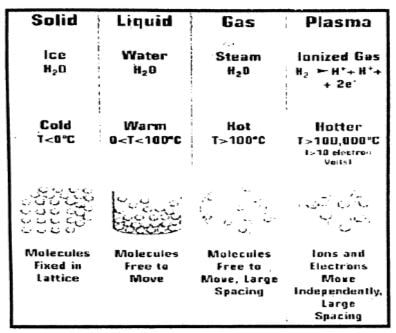##### USEFUL INFORMATION
 Density of various substance Substances Density in $kgm^{-1}$ Air 1.3 Foam 89 Petrol 800 Cooking Oil 920 Ice 920 Water 1000 Glass 2500 Aluminum 2700 Iron 7900 Copper 8900 Lead 11200 Mercury 13600 Gold 19300 Platinum 21500

##### USEFUL INFORMATION

1 metre cube (1 m3) = 1000 litre
1 litre = $10^{-3}$
1 cm3 = $10^{-6}$
1000 $kgm^{-3}$ = 1 $gcm^{-3}$

Does there exist a fourth state of matter? What is that?

Difficulty: Easy

Yes, the fourth state of matter is called plasma.

Plasma:

At a very high temperature, the collision between atoms a molecule tears off their actions. Atoms become positive ions. The ionic state of matter is called the plasma-the fourth state of matter.

What is meant by density? What is its SI unit?

Difficulty: Medium

Density:

The density of a substance is defined as its mass per unit volume.

Density =$\frac{(Mass of a substance)}{(volume of the substance)}$

OR

D=$\frac{m}{v}$

Unit of Density:

SI unit of density is kilogram per cubic meter $kgm^{-3}$).

Can we use a hydrometer to measure the density of milk?

Difficulty: Easy

Lactometer is used to measure the density of milk. Whereas hydrometer is used to measure the concentration of acid in a battery. It is called an acid meter.

Define the term pressure?

Difficulty: Easy

Pressure:

The force acting normally per unit area on the surface of a body is called pressure.

Thus

Pressure P  = $\frac{Force}{Area}$
Or                            P = $\frac{F}{A}$

Unit of Pressure:
In SI units, the unit of pressure is $Nm^{-2}$ also called pascal (Pa).

Thus
1 $Nm^{-2}$ = 1 Pa

Show that atmosphere exerts pressure.

Difficulty: Medium

Atmospheric Pressure:

The Earth is surrounded by a cover of air called the atmosphere. It extends to a few hundred kilometers above sea level. Just as certain sea creatures live at the bottom of the ocean, we live at the bottom of a huge ocean of air. Air is a mixture of gases. The density of air in the atmosphere is not uniform. It decreases continuously as we go up. Atmospheric pressure acts in all directions.

Experiment:

Take an empty tin can with a lid. Open its cap and put some water in it. Place it over the flame. Wait till the water begins to boil and the steam expels the air out of the can. Remove it from the flame. Close the can firmly by its cap. Now place the can under tap water. The can will squeeze due to atmospheric pressure.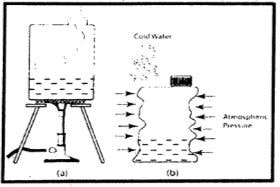When the can is cooled by tap water, the steam in it condenses. As the steam changes into water, it leaves a space behind it. This lowers the pressure inside the can as compared to the atmospheric pressure outside the can. This will cause the can to collapse from all directions. This experiment shows that the atmosphere exerts pressure in all directions.

The fact can also be demonstrated by collapsing of an empty plastic bottle when air is sucked out of it.

It is easy to remove air from a balloon but it is very difficult to remove air from a glass bottle why?

Difficulty: Easy

This is because the air inside the balloon is at fairly high pressure than the atmospheric pressure of the air outside the balloon. On the other hand air pressure inside the glass bottle is already equal to the atmospheric pressure so it is difficult to remove air from a glass bottle.

What is a barometer?

OR

Write the procedure for measuring atmospheric pressure with the help of a barometer?

Difficulty: Medium

Measuring Atmospheric Pressure:

At sea level, the atmospheric pressure is about 101,300 Pa or 101,300 $Nm^{-2}$.

Barometer:

The instruments that measure atmospheric pressure are called barometers. One of the simple barometers is a mercury barometer. It consists of a glass tube 1meter long closed at one end.

Construction:

After filling it with mercury, it is inverted in a mercury trough. Mercury in the tube descends and stops at a certain height. The column of mercury held in the tube exerts pressure at its base. At sea level, the height of the mercury column above the mercury in the trough is found to be about 76 cm. The pressure exerted by 76 cm of the mercury column is nearly 101,300 $Nm^{-2}$ equals atmospheric pressure. It is common to express atmospheric pressure in terms of the height of the mercury column. As the atmospheric pressure at a place does not remain constant, hence, the height of the mercury column also varies with atmospheric pressure.DO YOU KNOW

The fan in a vacuum cleaner lowers the air pressure in its bucket. The atmospheric air rushes into it carrying dust and dirt with it through its intake port. The dust and dirt particles are blocked by the filter while the air escapes out.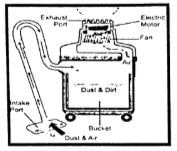Why water is not suitable to be used as the barometer?

Difficulty: Medium
Mercury is 13.6 times denser than water. Atmospheric pressure can hold a vertical column of water about 13.6 times the height of the mercury column at a place. Thus, at sea level, the vertical height of the water column would be $0.76 m \times 13.6$ = 10.34 m. Thus, a glass tube more than 10 m long is required to make a water barometer.

Following are the reasons why mercury and not water is used in a barometer:

i. Mercury is relatively denser than water, consequently, the length of the column of water would have to be about 34 feet high to exert pressure equal to that of the atmosphere while the column of mercury needs to be only 30 inches to exert pressure equal to that of pressure.

ii. Mercury has a very low vapor pressure when compared to that water. So, it is more sensitive than water to changes in atmospheric pressure and rises more quickly to record the changes in atmospheric pressure.

iii. Mercury’s freezing point is much lower than that of water so it can record the atmospheric pressure at temperatures below that of 0 degrees centigrade.

iv. Mercury does not evaporate easily so very little mercury vapor enters the vacuum above the mercury in the tube.

v. Mercury is a metal that shines brightly and so can be used to read the markings on the tube easily.

What makes a sucker press on a smooth wall to it?

Difficulty: Easy

The sucker is dish-shaped, when pressed against a smooth surface the air is forced beneath the sucker. The rubber makes an airtight seal and the air pressure outside is greater than the air pressure beneath the sucker, thus forcing the rubber sucker to ‘stick’.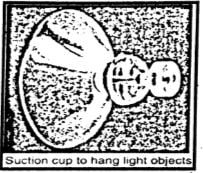Why does atmospheric pressure change with altitude?

Difficulty: Medium

Atmospheric pressure reduce with altitude for two reasons-both related to gravity.

• The gravitation attraction (g) between the earth and air molecule is greater for those molecules nearer to earth than those further away – they have more weight – dragging them close together and increasing the pressure (force per unit area) between them.
• Molecules further away from the earth have less weight (because gravitational attraction is less) but they are also ‘standing’ on the molecules below them, causing compression. The lower down have to support more molecules above them and are further compressed (pressurized) in the process.

Note:  It is gravitational force minus the effect of the Earth’s spin (an effect that is greatest at the equator)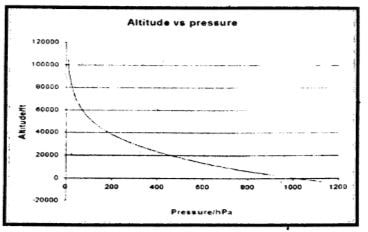DO YOU KNOW?

When air is sucked through a straw with its other end dipped in a liquid, the air pressure in the straw decreases. This causes the atmospheric pressure to push the liquid up the straw.

What does it mean when the atmospheric pressure at a place falls suddenly?

Difficulty: Easy

The changes in atmospheric pressure at a certain place indicate the expected changes in the weather conditions of that place. For example, a gradual and average drop in atmospheric pressure means a low pressure in a neighboring locality. A minor but rapid fall in atmospheric pressure indicates a windy and showery condition in the nearby region. A decrease in atmospheric pressure is accompanied by breeze and rain. Whereas a sudden fall in atmospheric pressure is often followed by a storm, rain, and typhoon to occur in a few hours.

What change is expected in the weather if the barometer reading shows a sudden increase?

Difficulty: Easy

On the other hand, increasing atmospheric pressure with a decline, later on, predicts an intense weather condition. A gradual large increase in the atmospheric pressure indicates a long spell of pleasant weather. A rapid increase in atmospheric pressure means that it will soon be followed by a decrease in atmospheric pressure indicating poor weather ahead.

State Pascal’s law.

Difficulty: Medium

Pascal’s law:

Pressure, applied at any point of a liquid enclosed in a container, is transmitted without loss to all other parts of the liquid.

Experiment:

It can be demonstrated with the help of a glass vessel having holes all over its surface.

Fill it with water. Push the piston. The water rushes out of the holes in the vessel with the same pressure. The force applied to the piston exerts pressure on the water. This pressure is transmitted equally throughout the liquid in all directions.

In general, this law holds good for fluids both liquids as well as gases.

Applications of Pascal’s law:

Pascal's law finds numerous applications in our daily life such as automobiles, hydraulic brake systems, hydraulic jacks, hydraulic presses, and other hydraulic machines.

Explain the working of a hydraulic press.

OR

A small force applied on the smaller piston results in a large force on the larger piston. Why?

Difficulty: Medium

Hydraulic Press:
A hydraulic press is a machine that works on Pascal's law. It consists of two cylinders of different cross-sectional areas. They have fitted with pistons of cross-sectional areas a and A.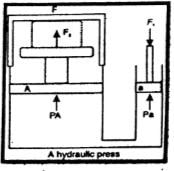The object to be compressed is placed over the piston of a large cross-sectional area A. The force $F_{1}$ is applied to the piston of the small cross-sectional area a. The pressure P produced by the small piston is transmitted equally to the large piston and a force $F_{2}$ acts on A which is much larger than $F_{1}$.
Pressure on the piston of the small area a is given by

P = $\frac{(F_{1})}{a}$
Applying Pascal's law, the pressure on the large piston of area A will be the same as on the small piston.
P  =$\frac{(F_{2})}{A}$

Comparing the above equations, we get
$\frac{(F_{1})}{a}$ = $\frac{(F_{2})}{A} F_{2}$ = $A \times \frac{(F_{1})}{a}$
Or, $F_{2}$ = $F_{1} \times\frac{(A)}{a}$  ………………..(i)

Notes: Since the ratio $\frac{(A)}{a}$ is greater than 1, hence the force $F_{2}$ that acts on the larger piston is greater than the force $F_{1}$ acting on the smaller piston. Hydraulic systems working in this way are known as force multipliers.

What is meant by elasticity?

Difficulty: Easy

Elasticity:

The property of a body to restore its original size and shape as the deforming force ceases to act is called elasticity.

Due to elasticity, we can determine the strength of a material and the deformation under the action of a force.

State Archimedes principle.

Difficulty: Hard

Then, $h_{2} – h_{1}$ = h
If $P_{1}$ and $P_{2}$ are the liquid pressures at depths $h_{1}$ and $h_{2}$ respectively and $\rho$ is its density, then according to the equation
$P_{1}$    =    $\rho g h_{1}$
$P_{2}$     =    $\rho g h_{2}$

Let the force $F_{1}$ be exerted at the cylinder top by the liquid due to pressure $P_{1}$ and the force $F_{2}$ is exerted at the bottom of the cylinder by the liquid due to $P_{2}$.

$F_{1}$ = $P_{1}$ A    =$\rho gh_{1}A$

And, $F_{2}$ = $P_{2}$ A = $\rho g$ $h_{2}$A
$F_{1}$ and $F_{2}$ are acting on the opposite faces of the cylinder.

Therefore, the net force F will be $F_{2}$ – $F_{1}$ in the direction of $F_{2}$. This net force F on the cylinder is called the upthrust of the liquid.
$F_{2} – F_{1}$ = $\rho g A (h_{2}- h_{1})$

Or, Up thrust of liquid = $\rho g (A h)$
(Ah = V)

Or, = $\rho g V$
= $( \rho V ) g$ $( \rho V = m )$
= mg         ……………..(i)

Here Ah is the volume V of the cylinder and is equal to the volume of the liquid displaced by the cylinder. Therefore,  $\rho gV$ is the weight of the liquid displaced. Equation (i) shows that an upthrust acts on the body immersed in a liquid and is equal to the weight of the liquid displaced, which is the Archimedes principle.

DO YOU KNOW?

Hydrometer:

A hydrometer is a glass tube with a scale marked on its stem and heavyweight in the bottom. It is partially immersed in a fluid, the density of which is to be measured. One type of hydrometer is used to measure the concentration of acid in a battery. It is called acid meter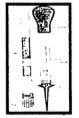What is upthrust? Explain the principle of floatation.

Difficulty: Medium

Upthrust:

Upthrust is a force that pushes an object up and makes it seem to lose weight in a fluid. (Remember, a fluid means a liquid or a gas).

The upthrust, or buoyancy, keeps ships afloat. The upthrust, or buoyancy, keeps swimmers on top of the water.

Principle of floatation:

An object sinks if its weight is greater than the upthrust acting on it. An object floats if its weight is equal to or less than the upthrust. When an object floats in a fluid, the upthrust acting on it is equal to the weight of the object.

In the case of a floating object, the object may be partially immersed. The upthrust is always equal to the weight of the fluid displaced by the object. This is the principle of floatation. It states that:

A floating object displaces a fluid having a weight equal to the weight of the object. Archimedes' principle applies to liquids as well as gases.

Explain how a submarine moves up the water surface down into the water.

Difficulty: Medium

Ships and submarines:

A submarine can travel over as well as underwater. It also works on the principle of floatation. It floats over water when the weight of water equal to its volume is greater than its weight. Under this condition, it is similar to a ship and remains partially above water level. It has a system of tanks that can be filled with and emptied from seawater. When these tanks are filled with seawater, the weight of the submarine increases. As soon as its weight becomes greater than the upthrust, it dives into the water and remains underwater. To come upon the surface, the tanks are emptied from seawater.

Why does a piece of the stone sink in water but a ship with huge weight floats?

Difficulty: Easy

It is due to Archimedes' principle. The density of the ship is less, it displaces more liquid, experiences more upward thrust, and floats whereas the density of stone is more, it displaces less liquid experiences less upward thrust, and sinks.

What is Hook’s law? What is meant by elastic limit?

Difficulty: Medium

Hook’s law:
The strain produced in a body by the stress applied to it is directly proportional to the stress within the elastic limit of the body.

Thus, $\text{stress} \propto \text{strain}$
Or, $\text{stress} = \text{constant} \times \text{strain}$
Or, $\frac{(\text{stress} )}{\text{strain}} = \text{constant}$

Elastic Limit:

The greatest stress that can be applied to a material without causing permanent deformation is called the elastic limit.

The stress point at which a material, if subjected to higher stress, will no longer return to its original shape. Brittle materials tend to break at or shortly past their elastic limit, while ductile materials deform at stress levels beyond their elastic limit.Take a rubber band. Construct a balance of your own using a rubber band. Check its accuracy by weighing various objects.

Difficulty: Medium

We know that the length of a rubber band increases on stretching it. Similarly, the pointer of a spring balance is lowered when a body is suspended from it. It is because the length of the spring inside the balance increases depending upon the weight of the suspended body.

A rubber band scale will be fairly accurate, but only for a short time. Eventually, the rubber band will begin to stretch and wear out. A better scale may be made by substituting a metal spring for the rubber band. Such a scale will be just as accurate, and because the spring is made of metal, it will last much longer.